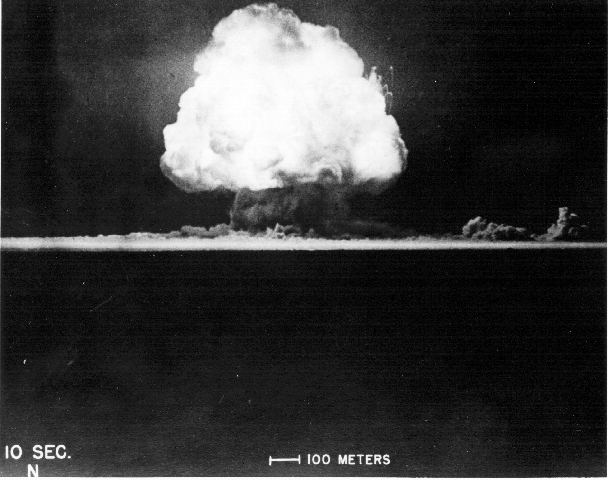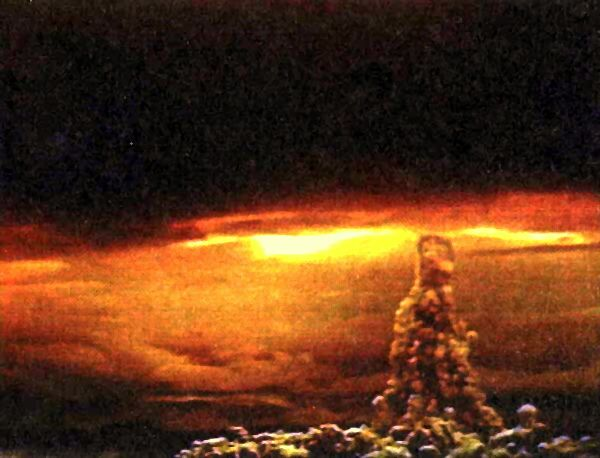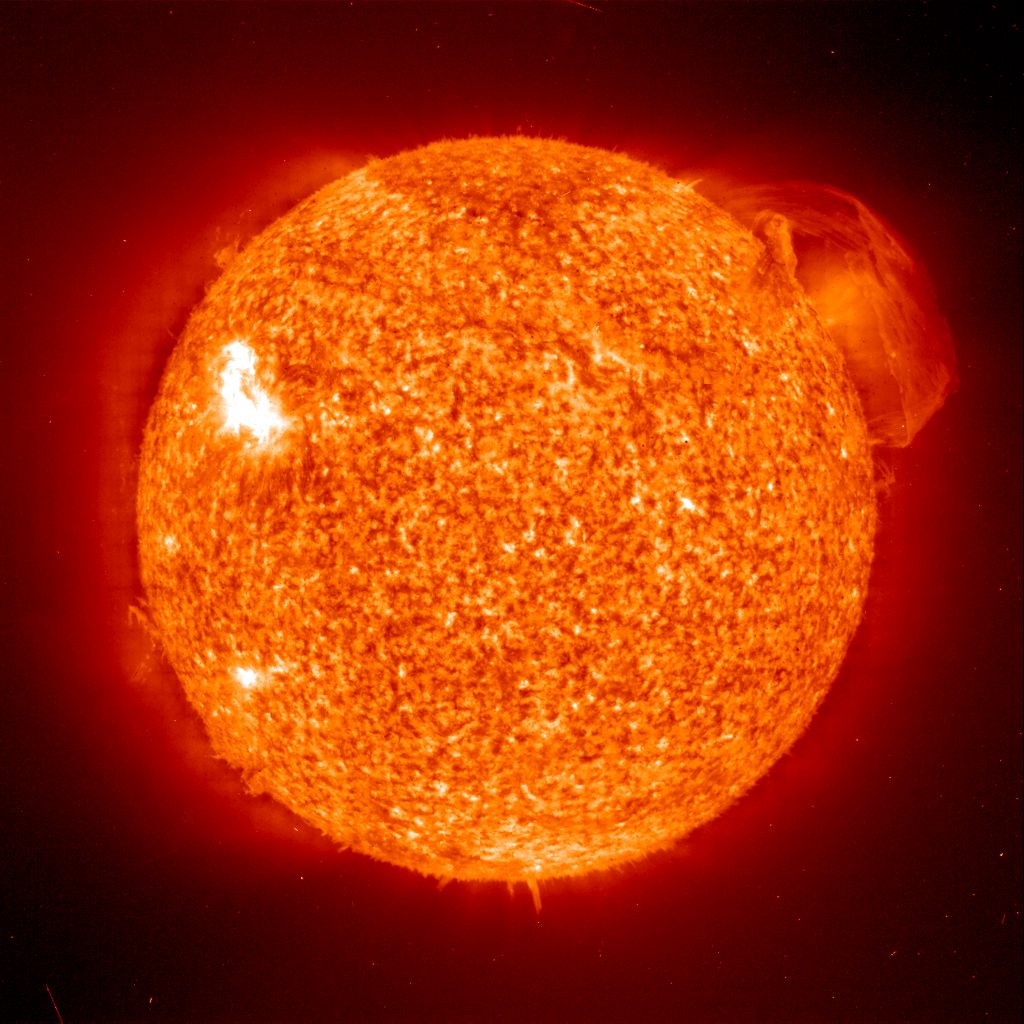Albert Einstein - A Centennial Celebration of His Miraculous Year
E=mc2 Supplement

On September 28, 1905 Einstein completed work on a manuscript he entitled "Does the Inertia of a Body Depend on Its Energy Content?" This small 3 page paper contains an afterthought to Einstein's work on special relativity. It is in this paper that he stated a version of his most famous equation,

E=mc2.

What this equation implies, is that energy and mass are two sides of the same coin. That is, energy can be converted into matter, and matter can be converted into energy. The meanings of the symbols are:

• E is energy (measured in joules),
• m is mass (measured in kilograms), and
• c is the speed of light in meters per second (so c=300,000,000 m/sec).
Since energy and mass are related by the constant of proportionality c2, which in terms of m2/sec2 is 9,000,000,000,000,000 (9 million billion), we see that a little amount of mass can be used to produce a tremendous amount of energy!

Unfortunately, it is very difficult to make this conversion. If we are not interested a controlled release of the energy, then there is one way to make the conversion...The Trinity bomb 10 seconds after detonation, from the Trinity Atomic Website.

On July 16, 1945 near Alamagordo, New Mexico at the "Trinity Site," the United States detonated an 18.6 kiloton bomb. The amount of energy released was equivalent to the conversion of 0.9 gram of matter into energy. One gram is roughly the mass of a standard paperclip.The Hiroshima bomb, from AtomicArchive.com.

The atomic bomb dropped on Hiroshima released an amount of energy equivalent to the conversion of 0.7 grams of matter into energy.The Tzar Bomba, from Wikipedia.
The largest nuclear bomb ever detonated was the Tsar Bomba which was exploded on October 30, 1961 by the Soviet Union. It released an amount of energy equivalent to the conversion of 2.3 kilograms of matter into energy. 2.3 kilograms is equal to about 5 pounds.

To get some grasp on the meaning of these numbers, let's consider the energy consumption of a typical Johnson City household. The Gardner household (consisting of 6 individuals) uses on average 2000 kilowatt hours per month. The amount of energy provided by the above mentioned atomic bombs would power this household for over 1000 years. The nuclear Tsar Bomba would power the Gardner household for over 2 million years.

 Trinity Hiroshima Nagasaki Tsar Bomba 1250 years 1000 years 1300 years 2.1 million yearsFrom NASA.
On a more peaceful note, it is Einstein's equation that explains how stars are able to burn so brightly for so many billions of years. Our own sun converts over 4 billion kilograms of matter into energy every second! Until Einstein explained this duality between mass and energy, the generation of something as simple as starlight was still a mystery.

A mathematical derivation of E=mc2 by Bob Gardner is available from a math seminar presentation entitled "E=mc2 Turns 100" in both PDF and PostScript formats.